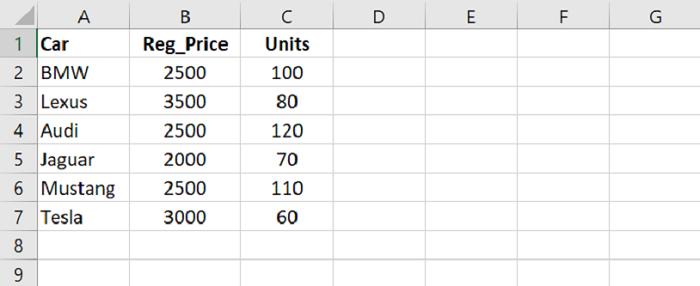# Python Pandas - Select a subset of rows from a dataframe

To select a subset of rows, use conditions and fetch data.

Let’s say the following are the contents of our CSV file opened in Microsoft Excel −At first, load data from a CSV file into a Pandas DataFrame −

dataFrame = pd.read_csv("C:\Users\amit_\Desktop\SalesData.csv")

Let’s say we want the Car records with “Units” more than 100 i.e. subset of rows. For this, use −

dataFrame[dataFrame["Units"] > 100]


Now, let’s say we want the Car records with “Reg_Price” less than 100 i.e. subset of rows. For this, use −

dataFrame[dataFrame["Reg_Price"] < 3000]

## Example

Following is the code −

import pandas as pd

# Load data from a CSV file into a Pandas DataFrame

# displaying two columns
res2 = dataFrame[['Reg_Price','Units']];
print("\nDisplaying two columns : \n",res2)

# selecting a subset of rows
print("\nSelect cars with Units more than 100: \n",dataFrame[dataFrame["Units"] > 100])

# selecting a subset of rows
print("\nSelect cars with Reg_Price less than 3000: \n",dataFrame[dataFrame["Reg_Price"] < 3000])

## Output

This will produce the following output −

Reading the CSV file...
Car   Reg_Price   Units
0      BMW        2500     100
1    Lexus        3500      80
2     Audi        2500     120
3   Jaguar        2000      70
4  Mustang        2500     110

Displaying only one column Car :
Reg_Price   Units
0        2500     100
1        3500      80
2        2500     120
3        2000      70
4        2500     110
Name: Car, dtype: object

Select cars with Units more than 100:
Car   Reg_Price   Units
2     Audi        2500     120
4  Mustang        2500     110

Select cars with Reg_Price less than 3000:
Car   Reg_Price   Units
0      BMW        2500     100
2     Audi        2500     120
3   Jaguar        2000      70
4  Mustang        2500     110